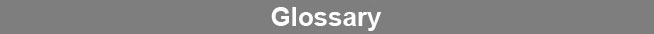Select Page### Gaussian Distribution

A Gaussian (normal) distribution is a symmetrical frequency distribution having a precise mathematical formula relating the mean and standard deviation of the samples. Gaussian distributions yield bell shaped frequency curves with a preponderance of values around the mean and progressively fewer observations as the curve extends outward.www.brendan.com